# Sweets

3 chocolate and 7 cakes cost 85, - CZK. 2 chocolates and 6 cakes cost 86, - CZK. How much is 5 chocolates and 9 cakes?

I wonder how to get the result, but only by logic without the use of a system of equations

Correct result:

x =  123

#### Solution:

3a + 7b = 85
6a + 2b = 86
5a + 9b = x

3•a + 7•b = 85
6•a + 2•b = 86
5•a + 9•b = x

3a+7b = 85
6a+2b = 86
5a+9b-x = 0

a = 12
b = 7
x = 123

Our linear equations calculator calculates it.We would be pleased if you find an error in the word problem, spelling mistakes, or inaccuracies and send it to us. Thank you!Tips to related online calculators
Do you have a system of equations and looking for calculator system of linear equations?

## Next similar math problems:

• Soccer balls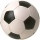Pupils in one class want to buy two soccer balls together. If each of them brings 12.50 euros, they will miss 100 euros, if each brings 16 euros, they will remain 12 euros. How many students are in the class?
• Clothes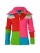CZK 2,400 cost clothes, a sweater by 150% than a T-shirt, trousers 2x more than a sweater, a jacket as a sweater and trousers together. What did the individual clothes cost?
• Fresh juiceThe seller offers fresh-squeezed juice, which the customer either pours into his own containers or sells it in liter plastic bottles, which the customer buys from him. A liter of juice costs 40 CZK more than a bottle. There is no charge for turning the ju
• Kohlrabies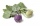The price of one kohlrabi increased by € 0.40. The number of kohlrabies that a customer can buy for € 4 has thus decreased by 5. Find out the new price of one kohlrabi in euros.
• Two teas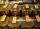A mixture weighing 10 kg was made from two types of tea. The price of tea was 160 CZK/kg and second tea 170 CZK/kg. The price of the mixture is 166 CZK/kg. How many kilograms of each type of tea had to be mixed?Adam has half the money in his right pocket than in his left pocket. If he transferred 40 crowns from the left pocket to the right, he would have the same in both pockets. Calculate how many crowns does Adam have in his left pocket more than in his right?
• The fourThe four pirates divided 65 coins to each other. They were sorted by age, the youngest receiving the least number of coins, each half more than the previous one. How many coins did the oldest pirate receive?
• StudentsStudents Aleš, Bohouš, Cyril, and Dušan were on a brigade. They divided the total revenue as follows: Aleš received two-fifths of the revenue, Bohouš received one-sixth of the revenue, Cyril received three-tenths of the revenue, and Dušan received the res
• ContestantsThe three best contestants are to divide the total prize of CZK 4,200. The second gets 20% more than the third. And the first one gets 200 CZK less than the second and the third together. How much will everyone get?
• Twelve ties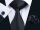The price of 12 ties before the discount was the same as 15 ties after discount. Calculate the price of 1 tie after discount, if you know that the discount price of one tie fell by 40 CZK?
• Wines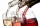Eleven liters of white wine and eight liters of red wine cost a total of 1315 kc. 1 liter of white wine was 10 kc cheaper than a liter of red wine. How much is 1 liter of white and how much red wine?
• Two accounts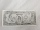Two accounts in the bank, one per year interest 2%, the second 3%. Total interest income 1900 USD. If interest rates were reversed, the yield would be USD 200 higher. What are the amounts on each account?
• Average monthly salaryA total of 10 teachers work at one small school in Moravia. The monthly salary of each is 21,500 CZK or 21,800 CZK or 22,500 CZK according to their education and age. The average monthly salary for this school's teacher is 21 850 CZK. How many teachers of
• Scholarship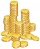The annual scholarship of the best student and second-best student in the class is 3500 euros in total. The best student scholarship in 8 months is the same as the second-best student scholar in the class for the whole year. How big is the annual scholars
• Trio ratioHans, Alena and Thomas have a total of 740 USD. Hans and Alena split in the ratio 5: 6 and Alena and Thomas in the ratio 4: 5. How much will everyone get?
• Aunt Rose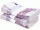Aunt Rose gave \$2500 to Mani and Cindy. Mani received \$500 more than Cindy. How mich did Cindy received?
• Dividing moneyVilem, Cenek, and Edita divided the money they earned by spreading the leaflet. Vilem got 240 CZK more than Cenek and twice more than Edita. Edita got 400kc less than Vilem.